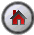SHAZAM Heteroskedasticity

## Estimation of Models with Heteroskedastic errors

With heteroskedastic errors the OLS estimator still gives an unbiased estimator. That is, the proof that the OLS estimator is unbiased does not use the heteroskedasticity assumption. The heteroskedasticity affects the results in two ways:

1. The OLS estimator is not efficient (it does not have minimum variance).
2. The estimators of the variances are biased. The standard errors reported on the SHAZAM output do not make any adjustment for the heteroskedasticity - so incorrect conclusions may be made if they are used in hypothesis tests.

A number of solution approaches can be considered as follows.

1.   The observed heteroskedasticity in the residuals may be an indication of model misspecification such as incorrect functional form. For example, a log-log model may reduce heteroskedasticity compared to a linear model. Gujarati [1995, p.386] comments that the log transformation compresses the scales in which the variables are measured.

2.   If the model specification is considered adequate then it may be useful to focus on just correcting the second problem above. The `HETCOV` option on the `OLS` command will compute White's (due to Hal White) heteroskedasticity-consistent covariance matrix of the parameter estimates. The SHAZAM OLS estimation output will then report the standard errors that are adjusted for heteroskedastic errors. These may be larger or smaller than the uncorrected standard errors. An application of heteroskedasticity-consistent standard errors is available.

3.   To obtain an efficient estimator an estimation method is weighted least squares (WLS). This is a special case of generalized least squares (GLS). The application of this method requires specifying a functional form for the error variance.

#### Examples of Weighted Least Squares

Note: Another estimation approach that has application to the estimation of models with heteroskedastic errors is maximum likelihood estimation. This is available in SHAZAM with the `HET` command. This command also has options for the estimation of time series models with ARCH and GARCH errors. Users must first study the principle of maximum likelihood as well as nonlinear optimisation methods before attempting to use the `HET` command.[SHAZAM Guide home]

### Computing Heteroskedasticity-Consistent Standard Errors

The `HETCOV` option on the `OLS` command is used to obtain standard errors that are corrected for some unknown form of heteroskedasticity.

#### Example

This example uses the Griffiths, Hill and Judge data set on household expenditure that was analyzed in the section on testing for heteroskedasticity. The SHAZAM commands (filename: `OLSHET.SHA`) below compute both the OLS standard errors and the heteroskedasticity corrected standard errors.

 ```SAMPLE 1 40 READ (GHJ.txt) FOOD INCOME OLS FOOD INCOME * Test for heteroskedasticity DIAGNOS / HET * Get the heteroskedasticity corrected standard errors OLS FOOD INCOME / HETCOV STOP ```

The SHAZAM output can be viewed. The OLS standard errors are reported in the following output:

 ``` VARIABLE ESTIMATED STANDARD T-RATIO PARTIAL STANDARDIZED ELASTICITY NAME COEFFICIENT ERROR 38 DF P-VALUE CORR. COEFFICIENT AT MEANS INCOME .23225 .5529E-01 4.200 .000 .563 .5631 .6871 CONSTANT 7.3832 4.008 1.842 .073 .286 .0000 .3129 ```

When the `HETCOV` option is specified the estimation results are:

 ``` VARIABLE ESTIMATED STANDARD T-RATIO PARTIAL STANDARDIZED ELASTICITY NAME COEFFICIENT ERROR 38 DF P-VALUE CORR. COEFFICIENT AT MEANS INCOME .23225 .6911E-01 3.361 .002 .479 .5631 .6871 CONSTANT 7.3832 4.292 1.720 .094 .269 .0000 .3129 ```

Both regressions report identical OLS estimated coefficients. But the second regression reports larger standard errors. So hypothesis testing that relies on the results of the first regression may give misleading results.[SHAZAM Guide home]

#### SHAZAM output

``` |_SAMPLE 1 40

UNIT 88 IS NOW ASSIGNED TO: GHJ.txt
2 VARIABLES AND       40 OBSERVATIONS STARTING AT OBS       1

|_OLS FOOD INCOME

OLS ESTIMATION
40 OBSERVATIONS     DEPENDENT VARIABLE = FOOD
...NOTE..SAMPLE RANGE SET TO:    1,   40

R-SQUARE =    .3171     R-SQUARE ADJUSTED =    .2991
VARIANCE OF THE ESTIMATE-SIGMA**2 =   46.853
STANDARD ERROR OF THE ESTIMATE-SIGMA =   6.8449
SUM OF SQUARED ERRORS-SSE=   1780.4
MEAN OF DEPENDENT VARIABLE =   23.595
LOG OF THE LIKELIHOOD FUNCTION = -132.672

VARIABLE   ESTIMATED  STANDARD   T-RATIO        PARTIAL STANDARDIZED ELASTICITY
NAME    COEFFICIENT   ERROR      38 DF   P-VALUE CORR. COEFFICIENT  AT MEANS
INCOME     .23225      .5529E-01   4.200      .000  .563      .5631      .6871
CONSTANT   7.3832      4.008       1.842      .073  .286      .0000      .3129

|_* Test for heteroskedasticity
|_DIAGNOS / HET

DEPENDENT VARIABLE = FOOD            40 OBSERVATIONS
REGRESSION COEFFICIENTS
0.232253330328       7.38321754308

HETEROSKEDASTICITY TESTS
CHI-SQUARE     D.F.   P-VALUE
TEST STATISTIC
E**2 ON YHAT:                     12.042     1    0.00052
E**2 ON YHAT**2:                  13.309     1    0.00026
E**2 ON LOG(YHAT**2):             10.381     1    0.00127
E**2 ON LAG(E**2) ARCH TEST:       2.565     1    0.10926
LOG(E**2) ON X (HARVEY) TEST:      4.358     1    0.03683
ABS(E) ON X (GLEJSER) TEST:       11.611     1    0.00066
E**2 ON X                 TEST:
KOENKER(R2):            12.042     1    0.00052
B-P-G (SSR) :           11.283     1    0.00078
E**2 ON X X**2    (WHITE) TEST:
KOENKER(R2):            14.582     2    0.00068
B-P-G (SSR) :           13.662     2    0.00108
|_STOP

|_* Get the heteroskedasticity corrected standard errors
|_OLS FOOD INCOME / HETCOV

OLS ESTIMATION
40 OBSERVATIONS     DEPENDENT VARIABLE = FOOD
...NOTE..SAMPLE RANGE SET TO:    1,   40

USING HETEROSKEDASTICITY-CONSISTENT COVARIANCE MATRIX

R-SQUARE =    .3171     R-SQUARE ADJUSTED =    .2991
VARIANCE OF THE ESTIMATE-SIGMA**2 =   46.853
STANDARD ERROR OF THE ESTIMATE-SIGMA =   6.8449
SUM OF SQUARED ERRORS-SSE=   1780.4
MEAN OF DEPENDENT VARIABLE =   23.595
LOG OF THE LIKELIHOOD FUNCTION = -132.672

VARIABLE   ESTIMATED  STANDARD   T-RATIO        PARTIAL STANDARDIZED ELASTICITY
NAME    COEFFICIENT   ERROR      38 DF   P-VALUE CORR. COEFFICIENT  AT MEANS
INCOME     .23225      .6911E-01   3.361      .002  .479      .5631      .6871
CONSTANT   7.3832      4.292       1.720      .094  .269      .0000      .3129
|_STOP
```